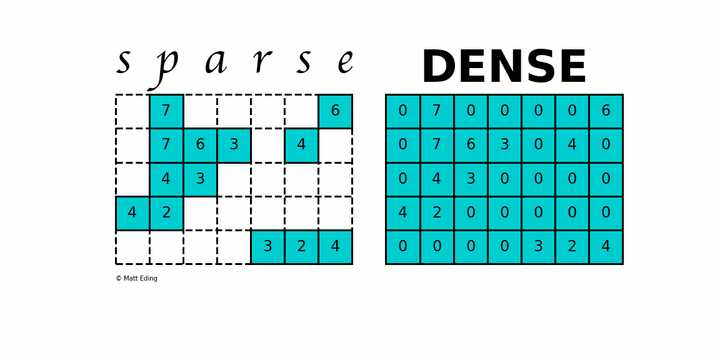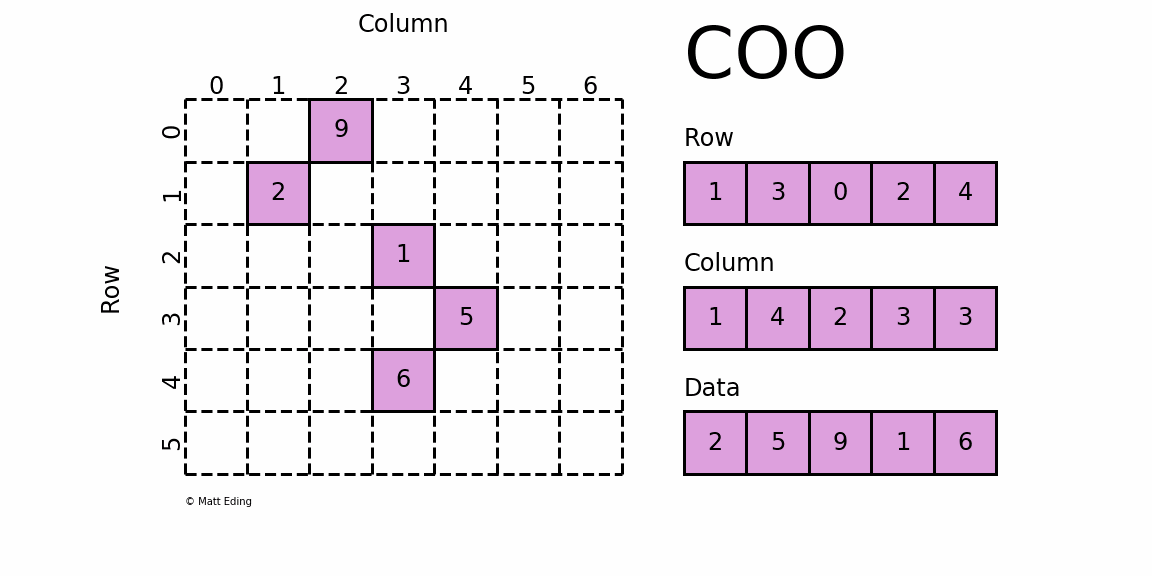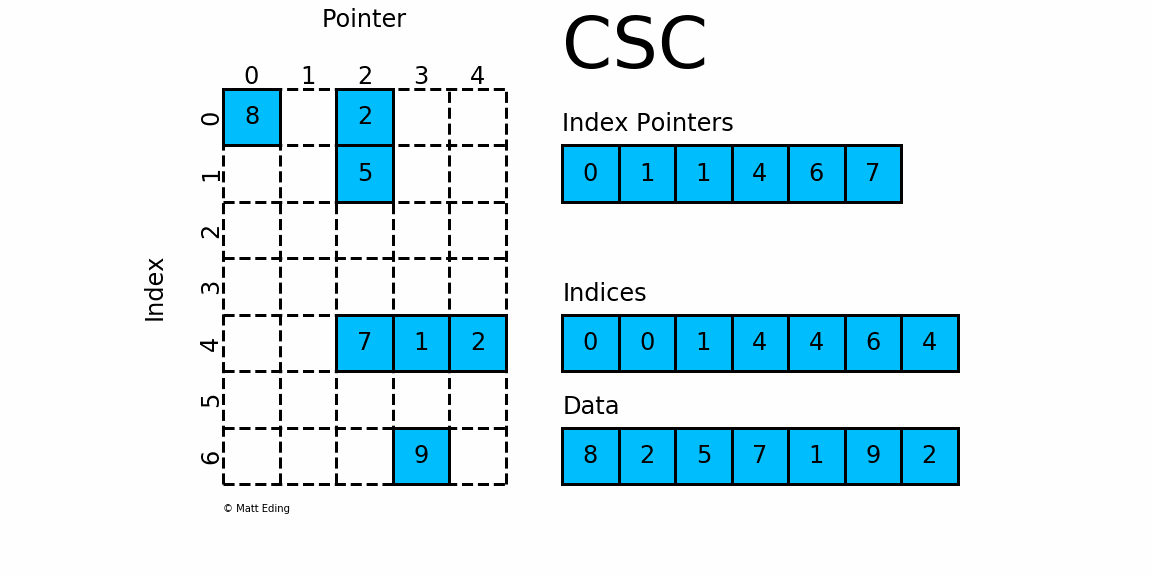# SciPy 稀疏矩阵 sparse matrix

spsolve(A, b)

https://docs.scipy.org/doc/scipy/reference/sparse.linalg.htmlSciPy 的 scipy.sparse 模块提供了处理稀疏矩阵的函数。

# 稀疏矩阵格式

- 高效修改 - 使用 DOK、LIL 或 COO。 这些通常用于构建矩阵。
- 有效的访问和矩阵操作 - 使用 CSR 或 CSC

## 1. coo_matrix

coo_matrix是最简单的稀疏矩阵存储方式（Coordinate list (COO)），采用三元组(row, col, data)(或称为ijv format)的形式来存储矩阵中非零元素的信息。在实际使用中，一般coo_matrix用来创建矩阵，但是因为coo_matrix无法对矩阵的元素进行增删改操作；创建成功一般转化成其他格式的稀疏矩阵（如csr_matrixcsc_matrix）进行转置、矩阵乘法等操作。coo_matrix可以通过四种方式实例化：
- coo_matrix(D), D代表密集矩阵；
- coo_matrix(S), S代表其他类型稀疏矩阵；
- coo_matrix((M, N), [dtype])构建一个shape为M*N的空矩阵，默认数据类型是d
- (row, col, data)三元组初始化。

##### 实例：

import numpy as np
from scipy.sparse import coo_matrix

_row  = np.array([0, 3, 1, 0])
_col  = np.array([0, 3, 1, 2])
_data = np.array([4, 5, 7, 9])
coo = coo_matrix((_data, (_row, _col)), shape=(4, 4), dtype=np.int32)
coo.todense()  # 通过toarray方法转化成密集矩阵(numpy.matrix)
matrix([[4, 0, 9, 0],
[0, 7, 0, 0],
[0, 0, 0, 0],
[0, 0, 0, 5]])
coo.toarray()  # 通过toarray方法转化成密集矩阵(numpy.ndarray)
array([[4, 0, 9, 0],
[0, 7, 0, 0],
[0, 0, 0, 0],
[0, 0, 0, 5]])


coo_matrix对象有以下属性：

print('矩阵中元素的数据类型:',coo.dtype)
print('获取矩阵的shape:',coo.shape)
print('获取矩阵的维度:',coo.ndim)
print('存储值的个数，包括显示声明的零元素(注意):',coo.nnz)


output:

矩阵中元素的数据类型: int32


print('稀疏矩阵存储的值，是一个1维数组:',coo.data)
print('与data同等长度的一维数组，表征data中每个元素的行号:',coo.row)
print('与data同等长度的一维数组，表征data中每个元素的列号:',coo.col)


output:

稀疏矩阵存储的值，是一个1维数组: [4 5 7 9]mmread()用于读取稀疏矩阵，mmwrite()用于写入稀疏矩阵，mminfo()用于查看稀疏矩阵文件元信息。(这三个函数的操作不仅仅限于coo_matrix)

from scipy.io import mmread, mmwrite, mminfo
HERE = dirname(__file__)
coo_mtx_path = join(HERE, 'data/matrix.mtx')
print(mminfo(coo_mtx_path))
# (13885, 1, 949, 'coordinate', 'integer', 'general')
# (rows, cols, entries, format, field, symmetry)
mmwrite(join(HERE, 'data/saved_mtx.mtx'), coo_mtx)


coo_matrix的优点：
- 有利于稀疏格式之间的快速转换（tobsr()tocsr()to_csc()to_dia()to_dok()to_lil()
- 允许又重复项（格式转换的时候自动相加）
- 能与CSR / CSC格式的快速转换

coo_matrix的缺点：
- 不能直接进行算术运算

## 2. csc_matrix 和 csr_matrix矩阵

• CSC - 压缩稀疏列（Compressed Sparse Column），按列压缩。
• CSR - 压缩稀疏行（Compressed Sparse Row），即按行压缩的稀疏矩阵存储方式。

### 2.1 csr_matrix

The compressed row storage (CRS) format puts the subsequent nonzeros of the matrix rows in contiguous memory locations. Assuming we have a nonsymmetric sparse matrix AAA, we create three vectors: one for floating point numbers (val) and the other two for integers (col_ind, row_ptr). The val vector stores the values of the nonzero elements of the matrix AAA as they are traversed in a row-wise fashion. The col_ind vector stores the column indexes of the elements in the val vector. That is, if val⁡(k)\=ai,j\operatorname{val}(\mathrm{k})=a_{i, j}val(k)\=ai,j, then col_ind (k)\=j(\mathrm{k})=j(k)\=j. The row_ptr vector stores the locations in the val vector that start a row; that is, if val⁡(k)\=ai,j\operatorname{val}(\mathrm{k})=a_{i, j}val(k)\=ai,j, then row_ptr (i)≤k<(i) \leq k<(i)≤k< row_ptr (i+1)(i+1)(i+1). By convention, we define row_ptr (n+1)\=nnz+1(\mathrm{n}+1)=n n z+1(n+1)\=nnz+1, where nnzn n znnz is the number of nonzeros in the matrix AAA. The storage savings for this approach is significant. Instead of storing n2n^2n2 elements, we need only 2nnz+n+12 n n z+n+12nnz+n+1 storage locations.csr_matrix可用于各种算术运算：它支持加法，减法，乘法，除法和矩阵幂,线性代数等操作。其有五种实例化方法：
- 通过密集矩阵构建；
- 通过其他类型稀疏矩阵转化；
- 构建一定shape的空矩阵；
- 通过(row, col, data)构建矩阵；
- csr_matrix((data, indices, indptr), [shape=(M, N)])
(https://blog.csdn.net/jeffery0207/article/details/100064602)

import numpy as np
from scipy.sparse import csr_matrix
A = np.array([0, 0, 0, 0, 0, 1, 1, 0, 2])
print(csr_matrix(A))


(0, 5)  1
(0, 6)  1
(0, 8)  2


• 第一行：在矩阵第一行（索引值 0 ）第六（索引值 5 ）个位置有一个数值 1。
• 第二行：在矩阵第一行（索引值 0 ）第七（索引值 6 ）个位置有一个数值 1。
• 第三行：在矩阵第一行（索引值 0 ）第九（索引值 8 ）个位置有一个数值 2。
>>> import numpy as np
>>> from scipy.sparse import csr_matrix

>>> indptr = np.array([0, 2, 3, 6])
>>> indices = np.array([0, 2, 2, 0, 1, 2])
>>> data = np.array([1, 2, 3, 4, 5, 6])
>>> csr = csr_matrix((data, indices, indptr), shape=(3, 3)).toarray()
array([[1, 0, 2],
[0, 0, 3],
[4, 5, 6]])



csr_matrix同样有很多方法，包括tobytes()，tolist(), tofile()，tostring()，csr_matrix对象属性前五个同coo_matrix，另外还有属性如下：

import numpy as np
from scipy.sparse import csr_matrix

indptr = np.array([0, 2, 3, 6])
indices = np.array([0, 2, 2, 0, 1, 2])
data = np.array([1, 2, 3, 4, 5, 6])
csr = csr_matrix((data, indices, indptr), shape=(3, 3))
print('密集矩阵转化的数组 csr.toarray():\n',csr.toarray())
print('每个元素对应的列号 csr.indices:',csr.indices)
print('矩阵每一行对应的所有之前行累计非零元素值 csr.indptr:',csr.indptr)
print('矩阵中的所有定义元素 csr.data:',csr.data)
print('判断每一行的indices列号是否是有序的，返回bool值:',csr.has_sorted_indices)


密集矩阵转化的数组 csr.toarray():
[[1 0 2]
[0 0 3]
[4 5 6]]



csr_matrix的优点

csr_matrix的缺点

## 2.2 csc_matrix## 2.3 lil_matrix

List of Lists format，又称为Row-based linked list sparse matrix。它使用两个嵌套列表存储稀疏矩阵：data保存每行中的非零元素的值，rows保存每行非零元素所在的列号(列号是顺序排序的)。这种格式很适合逐个添加元素，并且能快速获取行相关的数据。其初始化方式同coo_matrix初始化的前三种方式：通过密集矩阵构建、通过其他矩阵转化以及构建一个一定shape的空矩阵。lil_matrix可用于算术运算：支持加法，减法，乘法，除法和矩阵幂。其属性前五个同coo_matrix，另外还有rows属性，是一个嵌套List，表示矩阵每行中非零元素的列号。因此非常适合液体成型模拟，因为根据节点构建矩阵，方便通过行列修改元素。LIL matrix本身的设计是用来方便快捷构建稀疏矩阵实例，而算术运算、矩阵运算则转化成CSC、CSR格式再进行，构建大型的稀疏矩阵还是推荐使用COO格式。
lil_matrix优点：

lil_matrix缺点

## other matrix# scipy.sparse

identity(n[, dtype, format]) 生成稀疏单位矩阵
kron(A, B[, format]) sparse matrices A 和 B的克罗内克
kronsum(A, B[, format]) sparse matrices A 和 B的克罗内克
diags(diagonals[, offsets, shape, format, dtype]) 构建稀疏对角阵
spdiags(data, diags, m, n[, format]) 构建稀疏对角阵，同上，但不可指定shape
block_diag(mats[, format, dtype]) mats为iterable, 包含多个矩阵，根据mats构建块对角稀疏矩阵。
tril(A[, k, format]) 以稀疏格式返回矩阵的下三角部分
triu(A[, k, format]) 以稀疏格式返回矩阵的上三角部分
bmat(blocks[, format, dtype]) 从稀疏子块构建稀疏矩阵
hstack(blocks[, format, dtype]) 水平堆叠稀疏矩阵(column wise)
vstack(blocks[, format, dtype]) 垂直堆叠稀疏矩阵 (row wise)
rand(m, n[, density, format, dtype, …]) 使用均匀分布的值生成给定形状和密度的稀疏矩阵
random(m, n[, density, format, dtype, …]) 使用随机分布的值生成给定形状和密度的稀疏矩阵
eye(m[, n, k, dtype, format]) 生成稀疏单位对角阵（默认DIAgonal format

## data 属性查看存储的数据（不含 0 元素）：

import numpy as np
from scipy.sparse import csr_matrix
arr = np.array([[0, 0, 0], [0, 0, 1], [1, 0, 2]])
print(csr_matrix(arr).data)


[1 1 2]


## 使用 count_nonzero() 方法计算非 0 元素的总数：

import numpy as np
from scipy.sparse import csr_matrix
arr = np.array([[0, 0, 0], [0, 0, 1], [1, 0, 2]])
print(csr_matrix(arr).count_nonzero())


3


## 使用 eliminate_zeros() 方法删除矩阵中 0 元素：

import numpy as np
from scipy.sparse import csr_matrix
arr = np.array([[0, 0, 0], [0, 0, 1], [1, 0, 2]])
mat = csr_matrix(arr)
mat.eliminate_zeros()
print(mat)


  (1, 2)    1
(2, 0)    1
(2, 2)    2


## 使用 sum_duplicates() 方法来删除重复项:

import numpy as np
from scipy.sparse import csr_matrix
arr = np.array([[0, 0, 0], [0, 0, 1], [1, 0, 2]])
mat = csr_matrix(arr)
print(mat)
mat.sum_duplicates()
print(mat.todense())


  (1, 2)    1
(2, 0)    1
(2, 2)    2
[[0 0 0]
[0 0 1]
[1 0 2]]


## csr 转换为 csc 使用 tocsc() 方法：

import numpy as np
from scipy.sparse import csr_matrix
arr = np.array([[0, 0, 0], [0, 0, 1], [1, 0, 2]])
csr = csr_matrix(arr)
csc = csr.tocsc()
print('csr:',csr,'\n',csr.todense())
print('csc:',csc,'\n',csc.todense())


csr:
(1, 2)  1
(2, 0)  1
(2, 2)  2
[[0 0 0]
[0 0 1]
[1 0 2]]
csc:
(2, 0)  1
(1, 2)  1
(2, 2)  2
[[0 0 0]
[0 0 1]
[1 0 2]]